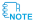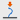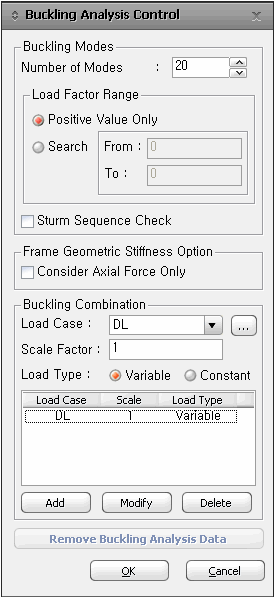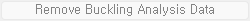Analysis Control Created Edited

# Buckling Analysis Control

## Function

• Enter the load cases and related data for the buckling analysis of a structure.
• midas Civil follows the procedure noted below for buckling analysis.

1. A buckling analysis process requires static analysis results to formulate the initial geometric stiffness matrix. Accordingly, enter the load cases to be applicable for the buckling analysis following the procedure for static analysis.

2. Invoke the dialog box of the Analysis > Analysis Control > Buckling Analysis Control menu and enter the required number of modes and data necessary for convergence and assign the load combination to be applied to the buckling analysis.

3. Select the Analysis > Perform Analysis menu or click Perform Analysis to carry out the analysis.

4. Once the analysis is successfully completed, use either the Results > Buckling Mode Shapes function or the Results > Result Tables > Bucking Mode Shape function to review the buckling mode shapes and critical load factors for each mode shape.Buckling analysis cannot be performed simultaneously with P-Delta analysis or eigenvalue analysis. Additionally, the elements that can be used for buckling analysis are limited to truss elements, beam elements (including the consideration of cross-sectional deformation), and plate elements.

## Call

From main menu, select  [Analysis] tab > [Analysis Control] group >[Buckling]

## Input### Buckling Modes

Number of Modes : Enter the number of buckling modes.

Positive Value Only : Produce the eigenvalue results only for the loading direction.Once buckling analysis is performed, buckling modes are computed for both the loading direction and the opposite direction. However, for static loads whose loading direction is fixed, it is not necessary to compute the buckling mode shape and factor for the opposite direction.

Search : Calculate the buckling load factor within the defined range

From : Minimum value of the buckling load factor

To : Maximum value of the buckling load factorWhen the reference value and the limit value are the same, the buckling load factors are calculated starting from the value closest to that reference value. In cases where the reference value and the limit value are different, the buckling load factors are calculated starting from the value closest to the reference value. However, only the values that exist between the reference value and the limit value are considered for calculation.

Sturm Sequence Check : Check to detect any missed buckling load factors. If there are any missed buckling load factors, an error message is displayed in the message window.

### Frame Geometric Stiffness Option

Consider Axial Force Only : Consider axial force only when formulating the geometric stiffness matrix.

### Buckling Combinations

Enter the load cases for the buckling analysis. Different Load Cases with the corresponding Scale Factors may be entered.

Load Type: In cases where non-increasing loads, such as self-weight, need to be considered in buckling analysis, it is used to incorporate such loads into the analysis.Strictly speaking, Constant should contain only the self-weight of the structure. However, it is common to apply dead load to slab, along with the self-weight. Accordingly, dead load can be entered as Constant Type.
To delete all the entered data, click.